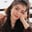RELATED TAGS

numpy

# What is logical_xor() in NumPy?Fouzia Bashir

## Overview

The logical_xor() method in NumPy is used to perform the element-wise logical exclusive OR operation. It returns a single boolean value or boolean ndarray.

The return value of a XOR operation is True between two values A and B when both input values are different. Otherwise, it returns False. Let's look at the truth table for XOR on two input values:

xor truth table
 A B A⊕B 0 0 0 0 1 1 1 0 1 1 1 0

### Syntax

numpy.logical_xor(x1,
x2,
/,
out=None,
*,
where=True,
casting='same_kind',
order='K',
dtype=None,
subok=True)
Signature

### Parameters

• x1, x2: Array-like objects are used to apply logical_xor. If x1 and x2 dimensions are not alike, they are broadcast to a standard shape.
• out: This is the location in memory used to store results. It can be ndarray or a tuple of ndarray or None.
• where: When a location becomes true, the out array is set to ufunc.
• casting: The default value for this is same_kind, meaning that object casting will be the same as float64 and float32.
• **kwargs: These are the keyword arguments.

### Return value

It returns a single boolean value or ndarray of boolean type.

### Example

In the code snippet below, we discuss different scenarios of the logical_xor() function where x1 and x2 can be either single boolean values or part of a boolean array. These values can be conditions where results are computed based on whether they're True or False.

# import numpy library in program
import numpy as np
# invoking logical xor on two boolean values
print("x1 & x2 as boolean values: ", np.logical_xor(True, False))
# invoking logical xor on two boolean arrays
print("x1 & x2 as boolean arrays: ", np.logical_xor([True, True, False, False], [True, False, True, False]))
# creating a numpy array from 0 to 10
x = np.arange(10)
# print logical_xor() results
print("x1 & x2 are conditions: ", np.logical_xor(x < 1, x > 3))

### Explanation

• Line 4: We calculate the logical XOR between two boolean values by calling np.logical_xor().
• Line 6: We calculate the logical XOR between two boolean arrays of length 4 by calling np.logical_xor() with arrays as arguments.
• Line 8–10: We calculate the logical XOR between two conditional values as x1 and x2 by calling np.logical_xor(). It returns a boolean array where each element of an array x satisfies the inequality x < 1 and x > 3.

RELATED TAGS

numpy

CONTRIBUTORFouzia Bashir
RELATED COURSES

View all Courses

Keep Exploring

Learn in-demand tech skills in half the time